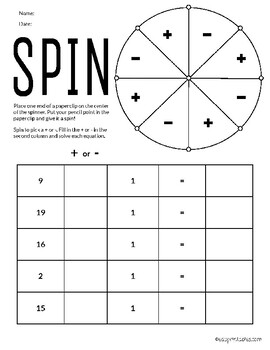# Spin! Addition and Subtraction Facts Using Numbers up to 20Subject
Resource Type
File Type

PDF

(2 MB|22 pages)
Standards
• Product Description
• StandardsNEW

Your students will practice their addition and subtraction facts using numbers up to 20 in this fun and interactive eduprintable activity.

Instructions:

Place one end of a paperclip on the center of the spinner. Put your pencil point in the paperclip and give it a spin!

Spin to pick a + or -. Fill in the + or - in the second column and solve each equation.

Determine the unknown whole number in an addition or subtraction equation relating three whole numbers. For example, determine the unknown number that makes the equation true in each of the equations 8 + ? = 11, 5 = ▯ - 3, 6 + 6 = ▯.
Understand subtraction as an unknown-addend problem. For example, subtract 10 – 8 by finding the number that makes 10 when added to 8.
Apply properties of operations as strategies to add and subtract. If 8 + 3 = 11 is known, then 3 + 8 = 11 is also known. (Commutative property of addition.) To add 2 + 6 + 4, the second two numbers can be added to make a ten, so 2 + 6 + 4 = 2 + 10 = 12. (Associative property of addition.)
Fluently add and subtract within 5.
For any number from 1 to 9, find the number that makes 10 when added to the given number, e.g., by using objects or drawings, and record the answer with a drawing or equation.
Total Pages
22 pages
N/A
Teaching Duration
N/A
Report this Resource to TpT
Reported resources will be reviewed by our team. Report this resource to let us know if this resource violates TpT’s content guidelines.